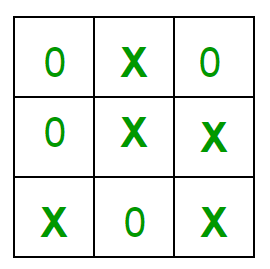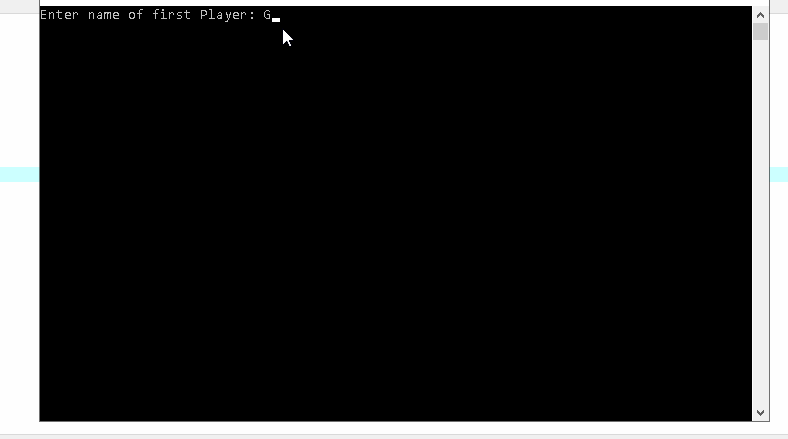Open in App
Not now

# Implementation of Tic-Tac-Toe for 2 person game (User vs. User)

• Difficulty Level : Medium
• Last Updated : 01 Aug, 2022

For 1-Person game (User vs. CPU), please refer Implementation of Tic-Tac-Toe game

Rules of the Game

• The game is to be played between two people (in this program between HUMAN to HUMAN).
• First the game will take the names of the two players as input.
• One of the player chooses ‘O’ and the other ‘X’ to mark their respective cells.
• There would be a game toss, which will decide which player will move first.
• The game starts with one of the players and the game ends when one of the players has one whole row/ column/ diagonal filled with his/her respective character (‘O’ or ‘X’).
• If the game is Tie then, A message is displayed “Game is Tie”.Winning Strategy – An Interesting Fact
If both the players play optimally then it is destined that you will never lose (“although the match can still be drawn”). It doesn’t matter whether you play first or second. In another way – “ Two expert players will always draw ”.
Isn’t this interesting?

Below is the code for the Game:

## C++

 `// C++ program to play Tic-Tac-Toe``#include ``using` `namespace` `std;` `// Length of the board``#define SIDE 3` `// Name of the players``string PLAYER1, PLAYER2;` `// Function to show the current``// board status``void` `showBoard(``char` `board[][SIDE])``{``    ``printf``(``"\n\n"``);` `    ``printf``(``"\t\t\t %c | %c | %c \n"``,``           ``board,``           ``board,``           ``board);` `    ``printf``(``"\t\t\t------------\n"``);` `    ``printf``(``"\t\t\t %c | %c | %c \n"``,``           ``board,``           ``board,``           ``board);` `    ``printf``(``"\t\t\t------------\n"``);``    ``printf``(``"\t\t\t %c | %c | %c \n\n"``,``           ``board,``           ``board,``           ``board);` `    ``return``;``}` `// Function to show the instructions``void` `showInstructions()``{``    ``printf``(``"\t\t\t Tic-Tac-Toe\n\n"``);` `    ``printf``(``"Choose a cell numbered "``           ``"from 1 to 9 as below"``           ``" and play\n\n"``);` `    ``printf``(``"\t\t\t 1 | 2 | 3 \n"``);``    ``printf``(``"\t\t\t------------\n"``);``    ``printf``(``"\t\t\t 4 | 5 | 6 \n"``);``    ``printf``(``"\t\t\t------------\n"``);``    ``printf``(``"\t\t\t 7 | 8 | 9 \n\n"``);` `    ``printf``(``"-\t-\t-\t-\t-\t"``           ``"-\t-\t-\t-\t-\n\n"``);` `    ``return``;``}` `// Function to initialise the game``void` `initialise(``char` `board[][SIDE],``                ``int` `moves[])``{``    ``// Initiate the random number``    ``// generator so that the same``    ``// configuration doesn't arises``    ``srand``(``time``(NULL));` `    ``// Initially the board is empty``    ``for` `(``int` `i = 0; i < SIDE; i++) {``        ``for` `(``int` `j = 0; j < SIDE; j++)``            ``board[i][j] = ``' '``;``    ``}` `    ``// Fill the moves with numbers``    ``for` `(``int` `i = 0; i < SIDE * SIDE; i++)``        ``moves[i] = i;` `    ``// randomise the moves``    ``random_shuffle(moves,``                   ``moves + SIDE * SIDE);` `    ``return``;``}` `// Function to declare winner of the game``void` `declareWinner(string whoseTurn)``{``    ``if` `(whoseTurn == PLAYER1)``        ``cout << PLAYER1 << ``" has won\n"``;``    ``else``        ``cout << PLAYER1 << ``" has won\n"``;``    ``return``;``}` `// Function that returns true if``// any of the row is crossed with``// the same player's move``bool` `rowCrossed(``char` `board[][SIDE])``{``    ``for` `(``int` `i = 0; i < SIDE; i++) {``        ``if` `(board[i] == board[i]``            ``&& board[i] == board[i]``            ``&& board[i] != ``' '``)``            ``return` `(``true``);``    ``}``    ``return` `(``false``);``}` `// Function that returns true if any``// of the column is crossed with the``// same player's move``bool` `columnCrossed(``char` `board[][SIDE])``{``    ``for` `(``int` `i = 0; i < SIDE; i++) {``        ``if` `(board[i] == board[i]``            ``&& board[i] == board[i]``            ``&& board[i] != ``' '``)``            ``return` `(``true``);``    ``}``    ``return` `(``false``);``}` `// Function that returns true if any``// of the diagonal is crossed with``// the same player's move``bool` `diagonalCrossed(``char` `board[][SIDE])``{``    ``if` `(board == board``        ``&& board == board``        ``&& board != ``' '``)``        ``return` `(``true``);` `    ``if` `(board == board``        ``&& board == board``        ``&& board != ``' '``)``        ``return` `(``true``);` `    ``return` `(``false``);``}` `// Function that returns true if the``// game is over else it returns a false``bool` `gameOver(``char` `board[][SIDE])``{``    ``return` `(rowCrossed(board)``            ``|| columnCrossed(board)``            ``|| diagonalCrossed(board));``}` `// Function to play Tic-Tac-Toe``void` `playTicTacToe(string whoseTurn)``{``    ``// A 3*3 Tic-Tac-Toe board for playing``    ``char` `board[SIDE][SIDE];` `    ``int` `moves[SIDE * SIDE];` `    ``// Initialise the game``    ``initialise(board, moves);` `    ``// Show instructions before playing``    ``showInstructions();` `    ``int` `moveIndex = 0, x, y;``    ``int` `r, c;` `    ``// Keep playing till the game is``    ``// over or it is a draw``    ``while` `(gameOver(board) == ``false``           ``&& moveIndex != SIDE * SIDE) {``        ``if` `(whoseTurn == PLAYER1) {` `        ``// Label for player1 wrong choice``        ``// of row and column``        ``player1:` `            ``// Input the desired row and``            ``// column by player 1 to``            ``// insert X``            ``cout << PLAYER1``                 ``<< ``" Enter the respective"``                 ``<< ``" row and column to "``                    ``"insert X :\n"``;``            ``cin >> r >> c;` `            ``if` `(r <= 3 && c <= 3) {` `                ``// To check desired row and``                ``// column should be empty``                ``if` `(board[r - 1] == ``' '``)``                    ``board[r - 1] = ``'X'``;` `                ``// If input is on already``                ``// filled position``                ``else` `{``                    ``cout << ``"You cannot Overlap"``                         ``<< ``" on Already "``                            ``"filled position:\n"``;``                    ``goto` `player1;``                ``}``            ``}` `            ``// Input is not valid``            ``else` `{``                ``cout << ``"\nInput is not "``                     ``<< ``"valid please enter "``                     ``<< ``"right one\n"``;` `                ``goto` `player1;``            ``}` `            ``showBoard(board);``            ``moveIndex++;``            ``whoseTurn = PLAYER2;``        ``}` `        ``else` `if` `(whoseTurn == PLAYER2) {` `        ``// Label for player2 wrong choice``        ``// of row and column``        ``player2:` `            ``// Input the desired row and``            ``// column by player 1 to``            ``// insert X``            ``cout << PLAYER2``                 ``<< ``" Enter the respective"``                 ``<< ``" row and column to "``                    ``"insert O :"``;``            ``cin >> r >> c;``            ``if` `(r <= 3 && c <= 3) {` `                ``// Input the desired row and``                ``// column by player 1 to``                ``// insert X``                ``if` `(board[r - 1] == ``' '``)``                    ``board[r - 1] = ``'O'``;` `                ``// If input is on already``                ``// filled position``                ``else` `{``                    ``cout << ``"You cannot Overlap"``                         ``<< ``" on Already "``                         ``<< ``"filled position:\n"``;``                    ``goto` `player2;``                ``}``            ``}` `            ``// Input is not valid``            ``else` `{``                ``cout << ``"\nInput is not "``                     ``<< ``"valid please enter "``                     ``<< ``"right one :\n"``;``                ``goto` `player2;``            ``}` `            ``showBoard(board);``            ``moveIndex++;``            ``whoseTurn = PLAYER1;``        ``}``    ``}` `    ``// If the game has drawn``    ``if` `(gameOver(board) == ``false``        ``&& moveIndex == SIDE * SIDE)``        ``printf``(``"It's a draw\n"``);``    ``else` `{` `        ``// Toggling the user to declare``        ``// the actual winner``        ``if` `(whoseTurn == PLAYER1)``            ``whoseTurn = PLAYER2;``        ``else` `if` `(whoseTurn == PLAYER2)``            ``whoseTurn = PLAYER1;` `        ``// Declare the winner``        ``declareWinner(whoseTurn);``    ``}``    ``return``;``}` `// Driver Code``int` `main()``{``    ``// Take the name of players``    ``cout << ``"Enter name of first Player: "``;``    ``cin >> PLAYER1;` `    ``cout << ``"Enter name of Second Player: "``;``    ``cin >> PLAYER2;` `    ``// Use current time as seed for``    ``// random generator``    ``srand``(``time``(0));` `    ``// Lets do toss``    ``int` `toss = ``rand``() % 2;` `    ``// Let us play the game``    ``if` `(toss == 1) {``        ``cout << ``"Player "``             ``<< PLAYER1``             ``<< ``" win the toss"``             ``<< endl;` `        ``playTicTacToe(PLAYER1);``    ``}``    ``else` `{``        ``cout << ``"Player "``             ``<< PLAYER2``             ``<< ``" win the toss"``             ``<< endl;``        ``playTicTacToe(PLAYER2);``    ``}` `    ``return` `(0);``}`

Output:
Below is the output of the above code:My Personal Notes arrow_drop_up